# Back to Basics: formulas in Google Sheets

Today I'm going to bring Google Sheets formulas to the table. I'll start with elements they consist of, remind you how they are calculated, and tell a difference between plain and complex formulas.

Here is what you need to know:

## The essence of Google Sheets formulas

First things first – to build a formula, you need logical expressions and functions.

A function is a mathematical expression; each with its own name.

For Google Sheets to know you're about to enter a formula rather than a number or text, start entering an equal sign (=) to a cell of interest. Then, type the function name and the rest of the formula.

Tip. You can check a complete list of all functions available in Google Sheets here.

• cell references
• named data ranges
• numeric and textual constants
• operators
• other functions

## Types of cell references

Each function requires data to work with, and cell references are used to indicate that data.

To reference a cell, alphanumeric code is used – letters for columns and numbers for rows. For example, A1 is the first cell in column A.

There are 3 types of Google Sheets cell references:

• Relative: A1
• Absolute: \$A\$1
• Mixed (half relative and half absolute): \$A1 or A\$1

The dollar sign (\$) is what changes the reference type.

Once moved, relative cell references change according to the destination cell. For example, B1 contains =A1. Copy it to C2 and it will turn to =B2. Since it was copied 1 column to the right and 1 row below, all coordinates have increased in 1.

If formulas have absolute references, they won't change once copied. They always indicate one and the same cell, even if new rows and columns are added to the table or the cell itself is shifted someplace else.

 Original formula in B1 =A1 =A\$1 =\$A1 =\$A\$1 Formula copied to C2 =B2 =B\$1 =\$A2 =\$A\$1

Thus, to prevent references from changing if copied or moved, use absolute ones.

To switch between relatives and absolutes quickly, just highlight any cell reference and press F4 on your keyboard.

At first, your relative reference – A1 – will change into absolute – \$A\$1. Press F4 once again, and you'll get a mixed reference – A\$1. On the next button hit, you'll see \$A1. Another one will return everything to its original state – A1. And so on.

Tip. To change all references at once, highlight the entire formula and press F4

### Data ranges

Google Sheets uses not only single cell references but also groups of adjacent cells – ranges. They are limited by the upper left and bottom right cells. For instance, A1:B5 signals to use all cells highlighted in orange below: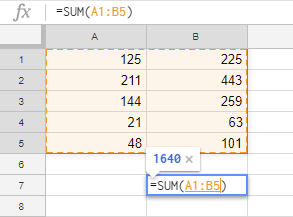## Constants in Google Sheets formulas

Constant values in Google Sheets are the ones that cannot be calculated and always remain the same. Most often, they are numbers and text, for example 250 (number), 03/08/2019 (date), Profit (text). These are all constants and we can alter them using various operators and functions.

For example, the formula may contain only constant values and operators:

`=30+5*3`

Or it can be used to calculate new value based on another cell's data:

`=A2+500`

Sometimes, though, you have to change the constants manually. And the easiest way to do that is to place each value into a separate cell and reference them in formulas. Then, all you need to do is make changes in a single cell rather than in all formulas.

So, if you put 500 to B2, refer to it with the formula:

`=A2+B2`

To get 700 instead, simply change the number in B2 and the result will be recalculated.

## Operators for Google Sheets formulas

Different operators are used in spreadsheets to preset the type and the order of calculations. They fall into 4 groups:

• arithmetic operators
• comparison operators
• concatenation operators
• reference operators

### Arithmetic operators

As the name suggests, these are used to perform math calculations such as adding, subtracting, multiplication, and division. As a result, we get numbers.

 Arithmetic operator Operation Example + (plus sign) Addition =5+5 - (minus sign) Subtraction Negative number =5-5 =-5 * (asterisk) Multiplication =5*5 / (slash) Division =5/5 % (percent sign) Percents 50% ^ (caret sign) Exponents =5^2

### Comparison operators

Comparison operators are used to compare two values and return a logical expression: TRUE or FALSE.

 Comparison operator Comparison condition Formula example = Equal to =A1=B1 > More than =A1>B1 < Less than =A1= More than or equal to =A1>=B1 <= Less than or equal to =A1<=B1 <> Not equal to =A1<>B1

### Text concatenation operators

Ampersand (&) is used to connect (concatenate) multiple text strings into one. Enter the below into one of Google Sheets cells and it will return Aircraft:

`="Air"&"craft"`

Or, put Surname to A1 and Name to B1 and get the Surname, Name text with the following:

`=A1&", "&B1`

### Formula operators

These operators are used to build Google Sheets formulas and indicate data ranges:

 Formula operator Action Formula example : (colon) Range operator. Creates reference to all cells between (and including) the first and the last cells mentioned. B5:B15 , (comma) Union operator. Joins multiple references into one. =SUM(B5:B15,D5:D15) (space) Intersection operator. References the intersection of two data ranges. B7:D7 C6:C8

All operators are of different priority (precedence) that defines the order of formula calculations and, most often, affects the resulting values.

## Order of calculations and operators precedence

Each formula in Google Sheets handles its values in some particular order: from left to right based on operator precedence. Operators of the same priority, e.g. multiplication and division, are calculated in the order of their appearance (left to right).

 Operators precedence Description : (colon) (space), (comma) Range operator - Minus sign % Percentage ^ Exponentiation * and / Multiplication and division + and - Addition and subtraction & Concatenate multiple textual strings into one =<><=>= Comparison

### How to use brackets to change the order of calculations

To change the order of calculations within the formula, enclose the part that should come first into brackets. Let's see how it works.

Suppose we have a standard formula:

`=5+4*3`

Since multiplication takes the lead and addition follows, the formula will return 17.

If we add brackets, the game changes:

`=(5+4)*3`

The formula adds numbers first, then multiplies them by 3, and returns 27.

The brackets from the next example dictate the following:

`=(А2+25)/SUM(D2:D4)`

• calculate value for A2 and add it to 25
• find the sum of values from D2, D3, and D4
• divide the first number to the sum of values

I hope it won't be difficult for you to get around these since we learn the order of calculations from a very young age and all arithmetics around us are performed this way. :)

## Named ranges in Google Sheets

Did you know you can label separate cells and entire data ranges? This makes processing large datasets quick and easy. Besides, you will guide yourself within Google Sheets formulas much faster.

Suppose you have a column where you calculate total sales per product and customer. Name such a range Total_Sales and use it in formulas.

I believe you would agree that the formula

`=SUM(Total_Sales)`

is far clearer and easier-to-read than

`=SUM(\$E\$2:\$E\$13)`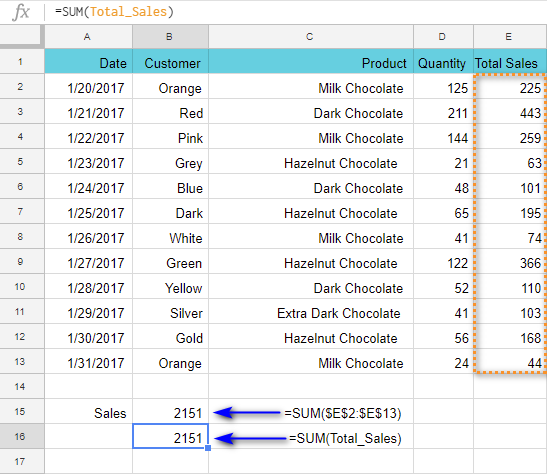Note. You can't create named ranges from non-adjacent cells.

To identify your range, do the following:

2. Go to Data > Named ranges in the sheet menu. A corresponding pane will appear on the right.
3. Set the name for the range and click Done.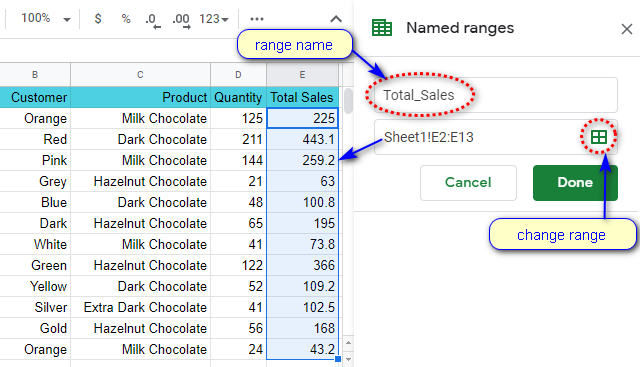Tip. This also lets you check, edit, and delete all ranges you've created: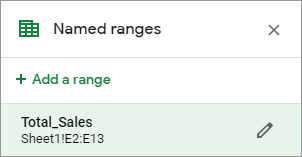### Picking correct name for the data range

Named ranges make your Google Sheets formulas friendlier, clearer, and understandable. But there's a small set of rules you should follow when it comes to labeling ranges. The name:

• Can contain only letters, numbers, underscores (_).
• Should not start from a number or from "true" or "false" words.
• Should not contain spaces ( ) or other punctuation marks.
• Should be 1-250 characters long.
• Should not tally with the range itself. If you try to name the range as A1:B2, the errors may occur.

If something goes wrong, e.g. you use space in the name Total Sales, you'll get an error right away. The correct name would be TotalSales or Total_Sales.

Note. Google Sheets named ranges are similar to absolute cell references. If you add rows and columns to the table, the Total_Sales range won't change. Move the range to any place of the sheet – and this won't alter the results.

## Types of Google Sheets formulas

Formulas can be simple and complex.

Simple formulas contain constants, references to cells on the same sheet, and operators. As a rule, it's either one function or an operator, and the order of calculations is very simple and straightforward – from left to right:

`=SUM(A1:A10)`
`=A1+B1`

As soon as additional functions and operators appear, or the order of calculations becomes a bit more complicated, the formula becomes complex.

Complex formulas may include cell references, multiple functions, constants, operators, and named ranges. Their length can be overwhelming. Only their author can "decipher" them quickly (but usually only if it was built not more than a week ago).

### How to read complex formulas with ease

There is a trick to make your formulas look comprehensible.

You can use as many spaces and line breaks as you need. This won't mess with the result and will arrange everything in the most convenient way.

To put a break line in the formula, press Alt+Enter on your keyboard. To see the entire formula, expand the Formula bar: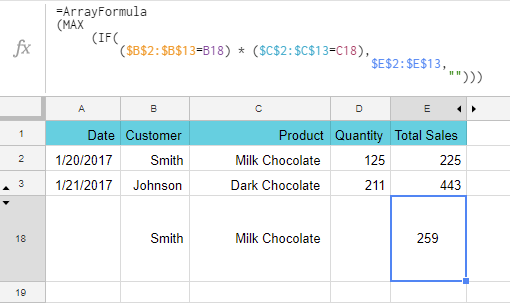Without these extra spaces and break lines, the formula would look like this:

`=ArrayFormula(MAX(IF((\$B\$2:\$B\$13=B18)*(\$C\$2:\$C\$13=C18), \$E\$2:\$E\$13,"")))`

Can you agree the first way is better?

Next time I'll dig deeper into building and editing Google Sheets formulas, and we'll practice a bit more. If you have any questions, please leave them in the comments below.

### 17 responses to "Back to Basics: formulas in Google Sheets"

1.Amy Flanders says:

thanks..that was super helpful...I have been trying to figure out absolute values for ever without knowing what I was looking for. It really clarified how sheets works..Cannot thank you enough!!
cheers
Amy

2.Shane Cammell says:

So I have a google sheet with multiple formulae in it. When I duplicate the sheet, the formuale don't work anymore. Help?

•Natalia Sharashova (Ablebits.com Team) says:

Hello Shane,

There are plenty of reasons for that, I'm afraid.

Perhaps, the references take too long to update, especially if there are too many formulas. It can be your internet connection speed/quality or a not-so-powerfull computer. You could also try and check the settings of the spreadsheet and its locale.

3.colin says:

Is it possible to count 1,2,3 in a single cell as 6. Say cell A1 has 123 in it and you want to add the numbers together in cell cell A2 to =6

•Natalia Sharashova (Ablebits.com Team) says:

Yes, it is, Colin. Try this:
=SUMPRODUCT(1*MID(A1,ROW(INDIRECT("1:"&LEN(A1))),1))

4.Phill says:

Hi there,
I'm relatively simple as far as spreadsheets go but have been battling with something that I am not sure can be achieved, here goes...

I have a simple finance spreadsheet that I use at home and I want to create a calculation that will be relative to a column that I delete each month, so it is replaced with the current month (there are 12 months in total).

Each time I delete the current column, I receive the #REF error in the calculation of the field that contains my calculation, seemingly because I have deleted the previous column. What I'm trying to do is fix the calculation so that it is clever enough to read the 'new ' column that has replaced the deleted one.

I have message around with different formulas but nothing seems to work, help!

•Natalia Sharashova (Ablebits.com Team) says:

Hi Phill,

I believe our Advanced Find and Replace will help you. By looking in formulas, it will find all references to the deleted column and change them all at once to the new reference. You can test the add-on for 30 days for free to see if it works as desired.

5.Andrew says:

I am working on a calculation that try's to create a number for say in cell D1, that takes a value input in say A1 subtracts a value in cell C1 from it, if the result if less than 0 , or minus ( ) , it reports 0, but if the number has a positive value , it then multiply's that number by cell E1 which is a percentage . For example
EXAMPLE 1 - A1 = 100 MINUS B1= 50 = D1 which would report 50 then X 11.5% would equal 5.75
HOWEVER EXAMPLE 2 A1 = 100 MINUS B1= 150 = D1 which would report (50) then X 11.5% would equal 0 . I THINK THIS IS A "IF" KIND OF CALCULATION, Any hints would be most welcome

•Natalia Sharashova (Ablebits.com Team) says:

Hello Andrew,

You're absolutely right, it's the IF function that is used to solve such tasks. You should be able to create the formula by following examples from this blog post: IF function in Google Sheets

6.Mike says:

I have multiple identical sheets with different values within a database. I want to create a summary sheet that would pull one line of data from each of the other sheets where the date field equals today. Any help with the formula?

7.Arch says:

=SUM(SUMIFS(AH11,H11,{"W","R","F","G"}))
In excel the above formula returns a value if WRF or G is found in H11
In google sheets it only returns a value for the first item, in this case "W"
How do I get sheets to return a value if any of the four letters are found in H11?

8.Randall says:

I am creating a spreadsheet for my cattle operation(which is small) I have cattle listed by tag color and number on tag. Is there a formula that I can apply to the spreadsheet that when I put in information in the form concerning a specific color for the ear tag and then a specific # value of the ear tag,It will populate in my spreadsheet in the proper corresponding cell range for that Cow I have listed for the color and # in my spreadsheet in sequence, without creating random entries at the bottom of the spreadsheet?

•Natalia Sharashova (Ablebits.com Team) says:

Hello Randall,

If I understand your task correctly, you could try using VLOOKUP or INDEX MATCH functions to pull responses returned by your form to whenever you need.

9.Isaac says:

I'm trying to see if there is a formula for adding A1 and B1 and A2 and B2 simply using the same formula for both equations? Can it be replicated for all A values and B values?

•Natalia Sharashova (Ablebits.com Team) says:

Hello Isaac,

I'm sorry but your task is not clear. For me to be able to suggest anything, please describe what you're trying to do in detail. Thank you.